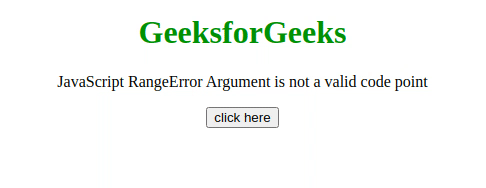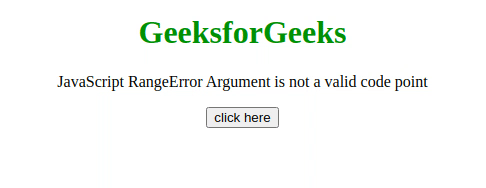Related Articles

# JavaScript RangeError Argument is not a valid code point

• Last Updated : 22 Jul, 2020

This JavaScript exception Invalid code point occurs if NaN values, negative Integers, non-Integers, or other values larger than 0x10FFFF are used with the String.fromCodePoint() method.

Message:

```RangeError: {0} is not a valid code point (Firefox)
RangeError: Invalid code point {0} (Chromium)
```

Error Type:

```RangeError

```

Cause of the error: The String.fromCodePoint() is used to return a string created using the sequence of code points that are specified as parameters. It throws this error if the passed code point values are NaN values, negative Integers, non-Integers, or values larger than 0x10FFFF.

Example 1: This example works without throwing any error because the value passed to the method is valid.

## HTML

 ```<``html``>``    ``<``head``>``        ``<``script` `src``=``"https://code.jquery.com/jquery-3.5.0.js"``>``        ````    ````    ``<``body` `style``=``"text-align: center;"``>``        ``<``h1` `style``=``"color: green;"``>``            ``GeeksforGeeks``        ```` ` `        ``<``p``>``            ``JavaScript RangeError ``            ``Argument is not a valid code point``        ```` ` `        ``<``button` `onclick``=``"Geeks();"``>``            ``click here``        ````        ``<``p` `id``=``"GFG_DOWN"``>`` ` `        ``<``script``>``            ``var el_down = document.getElementById("GFG_DOWN");``            ``function Geeks() {``                ``try {``                    ``String.fromCodePoint(34);``                    ``el_down.innerHTML = ``                      ``"'Argument is not a valid code point'" +``                      ``" error has not occurred";``                ``} catch (e) {``                   ` `                    ``// Show the error in console``                    ``console.log(e);``                    ``el_down.innerHTML = ``                      ``"'Argument is not a valid code point'" +``                      ``" error has occurred";``                ``}``            ``}``        ````    `````

Output:Example 2: In this example, the value passed to the method is NaN, which is an invalid value, therefore the error has occurred.

## HTML

 ```<``html``>``    ``<``head``>``        ``<``script` `src``=``"https://code.jquery.com/jquery-3.5.0.js"``>``        ````    ```` ` `    ``<``body` `style``=``"text-align: center;"``>``        ``<``h1` `style``=``"color: green;"``>``            ``GeeksforGeeks``        ```` ` `        ``<``p``>``            ``JavaScript RangeError ``            ``Argument is not a valid code point``        ```` ` `        ``<``button` `onclick``=``"Geeks();"``>``            ``click here``        ````        ``<``p` `id``=``"GFG_DOWN"``>`` ` `        ``<``script``>``            ``var el_down = document.getElementById("GFG_DOWN");``            ``function Geeks() {``                ``try {``                    ``String.fromCodePoint(NaN);``                    ``el_down.innerHTML = ``                      ``"'Argument is not a valid code point'" +``                      ``" error has not occurred";``                ``} catch (e) {``                    ``// Show the error in console``                    ``console.log(e);``                    ``el_down.innerHTML = ``                      ``"'Argument is not a valid code point'" +``                      ``" error has occurred";``                ``}``            ``}``        ````    `````

Output:Hey geek! The constant emerging technologies in the world of web development always keeps the excitement for this subject through the roof. But before you tackle the big projects, we suggest you start by learning the basics. Kickstart your web development journey by learning JS concepts with our JavaScript Course. Now at it’s lowest price ever!

My Personal Notes arrow_drop_up